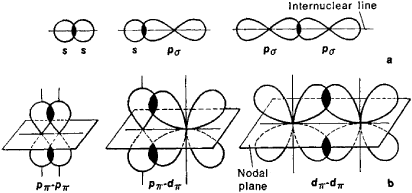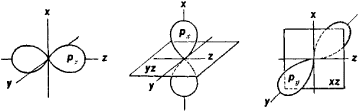Sigma and Pi Bonds

The following article is from The Great Soviet Encyclopedia (1979). It might be outdated or ideologically biased.

Sigma and Pi Bonds

(σ and π bonds), covalent chemical bonds characterized by a definite but varying spatial symmetry in the distribution of electron density. A covalent bond is formed as a result of the sharing of electrons between interacting atoms.

The resultant electron cloud of the σ bond is symmetrical about the line of the bond, that is, the line connecting the nuclei of the interacting atoms (Figure l,a). Single bonds in chemical compounds are usually σ bonds. The electron cloud of the π bond is symmetrical about the plane passing through the inter-nuclear line (Figure l,b), and the electron density in this plane, called the nodal plane, is equal to zero.Figure 1. Schematic representation of the spatial orientation of orbitals in the formation of (a) σ bonds as a result of s-s, s-ρσ, and Pσ-Pσ interaction and (b) π bonds as a result of ρπ-ρπ, ρπ-dπ, and dπ-dπ interaction

The use of the Greek letters σ and π is related to their correspondence to the Latin letters s and p. The letters s and p designate those electrons of the atom first able to participate in the formation of σ and π bonds, respectively. Inasmuch as the clouds of the atomic ρ orbitals (px, Py, Pz) are symmetrical about the corresponding Cartesian coordinate axes (x, y, z), if one ρ orbital, for example, pz, participates in the formation of a σ bond (the z-axis is the internuclear line), the two remaining p orbitals (px and py) may participate in the formation of two π bonds (their nodal planes are yz and xz, respectively; Figure 2). The d (Figure 1) and f electrons of an atom can also participate in the formation of σ and π bonds.Figure 2. Schematic representation of the px, py, and pz. electron clouds; the Cartesian coordinate axes and the nodal planes of the px and py orbitals are also shown

If both σ and π bonds are formed between atoms in a molecule, the resultant bond is called a multiple bond.

REFERENCES

Pimentel, G., and R. Spratley. Kak kvanlovaia mekhanika ob”iasniaet khimicheskuiu sviaz’. Moscow, 1973. (Translated from English.)
Shustorovich, E. M. Khimicheskaia sviaz’. Moscow, 1973.

E. M. SHUSTOROVICH